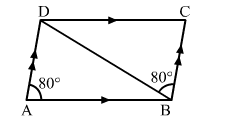# In the adjoining figure, ABCD is a parallelogram in which ∠DAB = 80° and ∠DBC = 60°.

Question:

In the adjoining figure, ABCD is a parallelogram in which DAB = 80° and ∠DBC = 60°. Calculate ∠CDB and ∠ADB.Solution:

Given:  ABCD is parallelogram and ∠DAB = 80°​ and ∠DBC = 60°
To find: Measure of ∠CDB and ∠ADB

In parallelogram ABCD, AD ||​ BC
∴ ∠DBC = ∠ ADB = 60o     (Alternate interior angles)     ...(i)

∴ ∠ADC = 180o  − ∠DAB
​    ⇒∠ADC​ = 180o − 80o = 100o